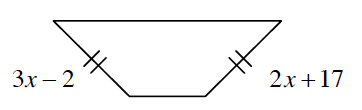### Home > CCG > Chapter 6 > Lesson 6.2.2 > Problem6-66

6-66.

Multiple Choice: Which equation below correctly represents the relationship of the sides given in the diagram at right?1. $3x-2+2x+17=360º$

1. $3x-2+2x+17=180º$

1. $3x-2+2x+17=90º$

1. $3x-2=2x+17$

Remember that sides with the same marks have the same length.

(d)